# Linear speed Formula (straight line motion)

Linear speed Formula (straight line motion)

Linear speed is the rate at which an object travels along a straight path. It is the distance an object travels in a certain amount of time. The units of linear speed are meters per second, m/s.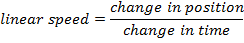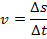v = linear speed (m/s)

Δs = short form for "the change in" position (m)

Δt = short form for "the change in" time (s)

Linear speed Formula(straight line motion) Questions:

1) A slug crawls across a garden 3.0 m wide in 5.0 minutes. What is the linear speed of the slug in meters per second?

Answer: The first step is to convert the amount of time the slug crawled for into seconds. There are 60 seconds in every minute.

Δt = 5.0 min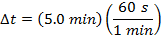Δt = 300 s

The next step is to use the formula to find the linear speed of the slug.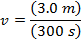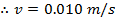The linear speed of the slug is 0.010 m/s.

2) An arrow is shot from a bow at a linear speed of 75.0 m/s. The target is 15.0 m from the bow. How much time passes between the arrow leaving the bow and when it hits the target?

Answer: The amount of time can be found by rearranging the formula for linear speed to solve for time.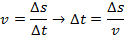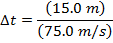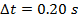The arrow leaves the bow, and reaches the target 0.20 seconds later.